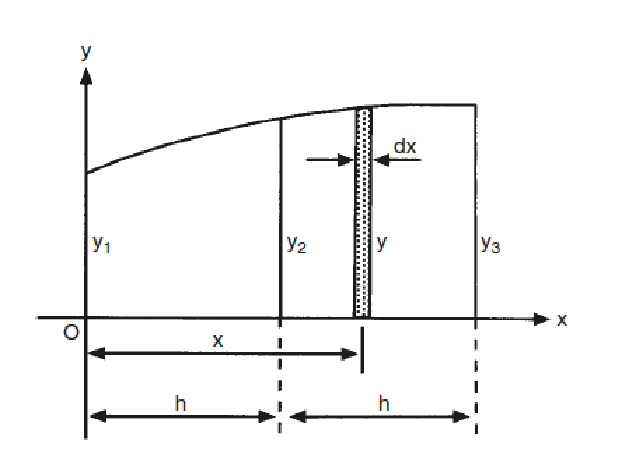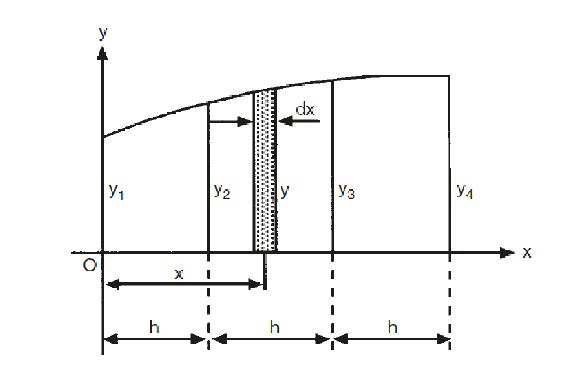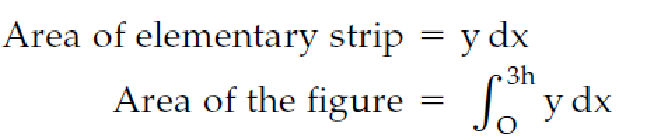# What is Simpson Rule and its details ?

Simpson’s Rules may be used to find the areas and volumes of irregular figures. The rules are based on the assumption that the boundaries of such figures are curves which follow a definite mathematical law. When applied to ships they give a good approximation of areas and volumes.

Simpson’s rule is a numerical method that approximates the value of a definite integral by using quadratic polynomials.

The accuracy of the answers obtained will depend upon the spacing of the ordinates and upon how near the curve follows the law.

Simpson’s First Rule:-

This rule assumes that the curve is a parabola of the second order. A parabola of the second order is one whose equation, referred to co-ordinate axes, is of the form .

y =  (a0 + a1x +  a2x2)

###### ln its simplest form, this rule states:-

The area between any three consecutive  ordinates is equal to the sum of  the end ordinates,plus four times the middle ordinate, all multiplied by one third of the common interval.Let the curve in Figure, be a parabola of the second order. Let y1, y2 and y3 be three ordinates equally spaced at ‘h’ units apart. The area of the elementary strip is y dx.Then the area enclosed by the curve and the axes of reference is given by:###### Simpson’s Second Rule:-

This rule assumes that the equation of the curve is of the third order, i.e. of a curve whose equation, referred to the co-ordinate axes, is of the form

y =  a0 +  a1x + a2x2 + a3x3,

###### where a0, a1, a2 and a3 are constants.

The area between any four consecutive ordinates  is  equal  to the sum of the end ordinates,plus threet imes eacho fthe middle ordinates, all multiplied by three-eighthso fthe common interval.